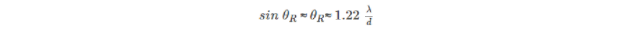• Call Now

1800-102-2727•

# Resolving Power of a Microscope

Microscopes and telescopes are essential components as they facilitate a better understanding of micro and macroscopic worlds. As the purpose of a microscope is to magnify the object’s size, the device contributes to several discoveries in physics, science, chemistry, biology, etc.

On the other hand, telescopes are used for viewing distant objects, and they produce an image that is larger than the image produced in the unaided eye. Thus, it is essential to know specific properties such as the resolution of microscope and telescope, etc. In this article, we discuss in detail the resolving power of a microscope and telescope.

Resolving power is defined as the capacity to see the objects located at a distance; It is known as the inverse of the distance or separation between two objects that can be just resolved when viewed through the optical instrument. For telescope and microscope, we can define the resolving power as their ability to distinguish between two firmly placed objects located at a distance and produce their images. Thus, more the resolving power is the ability of the optical instrument to distinguish and recognize the distance between two objects.

Let us assume you want to view stars using a telescope. However, whenever you see the stars, they appear blurred, or two stars may appear as one as they are very close to each other. However, these stars should be displayed as two separate stars. Thus, this ability of an optical device to display them as separate stars is called resolving power.

As the resolving power of an optical instrument is a ratio of a mean wavelength of a pair of spectral lines to the difference between the wavelength, i.e., both are the exact quantities, it has no unit.

However, the resolving power of optical instruments is limited by diffraction or aberration. While diffraction occurs whenever light passes through an obstacle, aberration is defined as a problem of geometrical optics. Due to aberration and diffraction, the circular aperture of optical lenses produces a diffraction pattern of concentric rings that grow fainter as we move away from the center. Due to this, a blurred image is produced. So diffraction limits the resolution of any system having a lens or mirror. Telescopes are also limited by diffraction because of the finite diameter of their primary mirror.

## Rayleigh’s criterion

Hence, to resolve the problem of blurred images, the diffraction of light should be avoided. This can be avoided by defining the minimum distance between images. According to Rayleigh's criterion for the diffraction limit to resolution, two images are just resolvable when the center of the diffraction pattern of one is directly over the first minimum of the diffraction pattern of the other. The formula gives the criterion.## Resolving power of a telescope

Based on the uses and applications of a telescope, we require large apertures to resolve the power of telescopes. In order to evaluate the resolving formula for the telescope, we use Rayleigh's criterion formula. Based on Rayleigh's formula, the angular separation between two distant objects should be

Resolving Power = D/d = a/1.22λ

Where,
a = width of the rectangular slit
D = distance of objects of the telescope.
Hence, if the diameter d is greater, the resolution of the telescope will be better. The astronomical and optical telescopes usually consist of a mirror of large diameters larger than 10m to get the desired resolution. Large wavelengths help reduce the resolving power of the telescope, and hence the radio and microwave telescopes require larger mirrors.

## Resolving power of Microscope

Microscopes are often used for scientific analysis or research. Thus, the resolving power of a microscope is inversely proportional to the distance between the two objects. In 1873, Earnest Abbe put forth the formula for resolving the power of a microscope. According to Earnest Abbe's criterion, the resolution of a microscope R depends on the angular aperture.Where NA = n sinθ
NA = the numerical aperture,
θ = half of the angle α of the lens, which depends on the focal length and the diameter of the lens,
n = refractive index of the medium between the specimen and the lens, and λ = wavelength of light that is emerging from the object under consideration.
It has to be noted that the diffraction of an aperture restricts the resolving power of the light microscope. Therefore, for a resolution to occur, the first-order diffracted beam & direct beam must be collected as an objective of the microscope.Talk to our expert
Resend OTP Timer =
By submitting up, I agree to receive all the Whatsapp communication on my registered number and Aakash terms and conditions and privacy policy